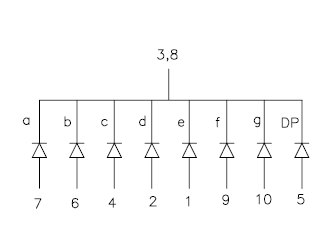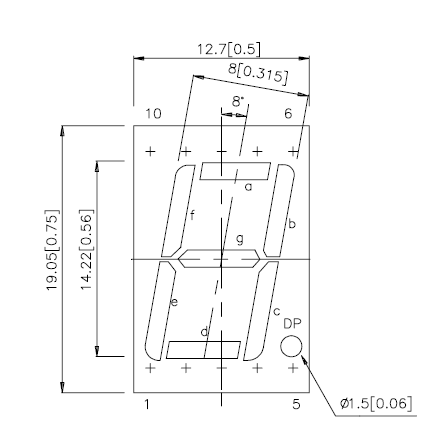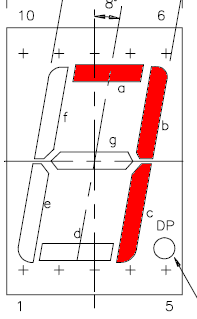# Controlling 7 segment LED display from Arduino. Full code included.

So this is first real electronics mini project/tutorial in this blog.

Everybody at the beginning usually shows how to turn on one LED,  I do it a little more complex by controlling 7 segment LED display.

For this demonstration I use one Kingbright SC56-11EWA module with common cathode, it means that all 8 (7 segments +  1 dot) LED’s cathode are connected together:The first step is to connect 7 segment display LED’s anodes to Arduino ports. You need 7 free ports, or 8 if You want use “dot”. Display datasheet comes very handy, when You need to found out which pin is which.Do not forget to connect LED’s pins with resistors in serial. Typical red LED forward voltage is 2.0V @20mA current, but Arduino ports outputs 5V, so You must use resistor, in this situation (5-2)/0.02= 150 Ohm or higher should be ok.

Common cathode (3 and/or 8 pin) should be connected to GND and if You have display with common anode You should connect it to +5V.

So if everything is connected we should start to analyze program code.

To make life easier I have done same definitions, so after that I don’t need to remember which Arduino ports I selected to connect display’s segments:

```#define G 22 #define F 23 #define A 24 #define B 25 #define E 26 #define D 27 #define C 28 #define DP 29```

Arduino ports can be outputs (e.g. for controlling LED) or inputs (e.g. to detect pressed button, sense voltage). We need outputs to turn on LED’s so:

```pinMode(A, OUTPUT); pinMode(B, OUTPUT); pinMode(C, OUTPUT); pinMode(D, OUTPUT); pinMode(E, OUTPUT); pinMode(F, OUTPUT); pinMode(G, OUTPUT); pinMode(DP, OUTPUT);```

To display digit on display we need to turn on some segments accordingly to digit.

For example for “7” we need to turn on A B C segments and other segments should be turned off.So where is function for what as and for all other digits:
`void digit7 () {`

` `

```digitalWrite(A,HIGH); digitalWrite(B, HIGH); digitalWrite(C, HIGH); digitalWrite(D, LOW); digitalWrite(E, LOW); digitalWrite(F, LOW); digitalWrite(G, LOW); }; ```

I also have made a function to display digit form variable, it is very useful in main loop.

```void showdigit (int digit) { switch (digit) { case 0: digit0 (); break; ... case 9: digit9 (); break; default: break; };```

And  the main program. Using for loop as counter from 0 to 9. You see how `showdigit` function is good here, it shows variable “i” content on display.

```for (int i=0;i<10;i++) { //counting from 0 to 9 showdigit(i); delay (1000); // 1000ms= 1s delay if (i%2) { digitalWrite(DP, HIGH); } else {digitalWrite(DP, LOW); };```

If condition is here to check if variable “i” is even and if so shows dot on the display.

Completed code:

```/* 7 LED segments demo counter program from electronicsblog.net. If You share/use this code elsewhere on the internet please mention this code source. */```

`// segment | Arduino board PIN number`

``` #define G 22 #define F 23 #define A 24 #define B 25 #define E 26 #define D 27 #define C 28 #define DP 29 // functions to display digits void digit0 () { // for 0 needed to turn ON F A B C D E segments, so: digitalWrite(A, HIGH); digitalWrite(B, HIGH); digitalWrite(C, HIGH); digitalWrite(D, HIGH); digitalWrite(E, HIGH); digitalWrite(F, HIGH); //////////////////////// G segment should be turn OFF digitalWrite(G, LOW); }; void digit1 () { digitalWrite(A,LOW); digitalWrite(B, HIGH); digitalWrite(C, HIGH); digitalWrite(D, LOW); digitalWrite(E, LOW); digitalWrite(F, LOW); digitalWrite(G, LOW); }; void digit2 () { digitalWrite(A,HIGH); digitalWrite(B, HIGH); digitalWrite(C, LOW); digitalWrite(D, HIGH); digitalWrite(E, HIGH); digitalWrite(F, LOW); digitalWrite(G, HIGH); }; void digit3 () { digitalWrite(A,HIGH); digitalWrite(B, HIGH); digitalWrite(C, HIGH); digitalWrite(D, HIGH); digitalWrite(E, LOW); digitalWrite(F, LOW); digitalWrite(G, HIGH); }; void digit4 () { digitalWrite(A,LOW); digitalWrite(B, HIGH); digitalWrite(C, HIGH); digitalWrite(D, LOW); digitalWrite(E, LOW); digitalWrite(F, HIGH); digitalWrite(G, HIGH); }; void digit5 () { digitalWrite(A,HIGH); digitalWrite(B, LOW); digitalWrite(C, HIGH); digitalWrite(D, HIGH); digitalWrite(E, LOW); digitalWrite(F, HIGH); digitalWrite(G, HIGH); }; void digit6 () { digitalWrite(A,HIGH); digitalWrite(B, LOW); digitalWrite(C, HIGH); digitalWrite(D, HIGH); digitalWrite(E, HIGH); digitalWrite(F, HIGH); digitalWrite(G, HIGH); }; void digit7 () { digitalWrite(A,HIGH); digitalWrite(B, HIGH); digitalWrite(C, HIGH); digitalWrite(D, LOW); digitalWrite(E, LOW); digitalWrite(F, LOW); digitalWrite(G, LOW); }; void digit8 () { digitalWrite(A, HIGH); digitalWrite(B, HIGH); digitalWrite(C, HIGH); digitalWrite(D, HIGH); digitalWrite(E, HIGH); digitalWrite(F, HIGH); digitalWrite(G, HIGH); }; void digit9 () { digitalWrite(A, HIGH); digitalWrite(B, HIGH); digitalWrite(C, HIGH); digitalWrite(D, HIGH); digitalWrite(E, LOW); digitalWrite(F, HIGH); digitalWrite(G, HIGH); }; //function to display digit from inputed int void showdigit (int digit) { switch (digit) { case 0: digit0 (); break; case 1: digit1 (); break; case 2: digit2 (); break; case 3: digit3 (); break; case 4: digit4 (); break; case 5: digit5 (); break; case 6: digit6 (); break; case 7: digit7 (); break; case 8: digit8 (); break; case 9: digit9 (); break; default: break; }; } void setup() { pinMode(A, OUTPUT); pinMode(B, OUTPUT); pinMode(C, OUTPUT); pinMode(D, OUTPUT); pinMode(E, OUTPUT); pinMode(F, OUTPUT); pinMode(G, OUTPUT); pinMode(DP, OUTPUT); }; void loop () { for (int i=0;i<10;i++) { //counting from 0 to 9 showdigit(i); delay (1000); // 1000ms= 1s delay if (i%2) { digitalWrite(DP, HIGH); } else {digitalWrite(DP, LOW); }; }; ```

`};`